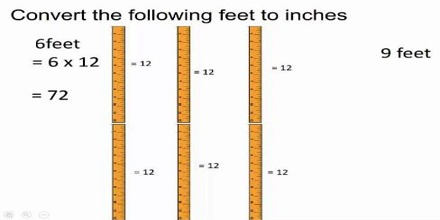Subtracting Feet and Inches

Handles operations for feet whole numbers and inches in mixed numbers for adding, subtracting, dividing or multiplying whole numbers, fractions or mixed numbers and returns an answer in a reduced fraction and a mixed number, if it exists.

When you begin learning about measurements in math class, one of the first things you learn is that there are 12 inches in a foot. When you are faced with a math problem that requires you to subtract feet and inches, you might get confused because they aren’t the same numbers. This type of problem will require you to deal with the inches and feet separately. Not only do you need skills in subtracting, but depending on the problem, you might have to use adding and reasoning skills as well.If the number of inches being subtracted is greater than the number of inches in the length that is being subtracted from, decrease the number of feet by one and increase the number of inches by 12 in the length from which you are subtracting.

• Subtract the inches.
• Subtract the feet.

Example: Subtract 3 feet 10 inches from 6 feet 8 inches.

The number of inches being subtracted is larger so adjust the number of inches:

6 feet 8 inches = 5 feet 20 inches

Subtract the inches: 20 – 10 = 10 inches

Subtract the feet: 5 – 3 = 2 feet